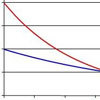#### You may also like### Scale Invariance

By exploring the concept of scale invariance, find the probability that a random piece of real data begins with a 1.### Into the Exponential Distribution

Get into the exponential distribution through an exploration of its pdf.### PCDF

When can a pdf and a cdf coincide?

# PDF

##### Age 16 to 18 Challenge Level:
The area under the graph of the probability density function between $x=a$ and $x=b$ gives the probability that the outcome is between $a$ and $b$ so the total area under the graph must be $1$, in this example for $x$ between $0$ and $3$.

To find the median we have to find the value $t$ such that the area under the graph for $0\leq x \leq t$ is $0.5$. You will have to find the roots of a cubic equation (which you should be able to factorise) in this example and then identify the root which lies in the required interval.